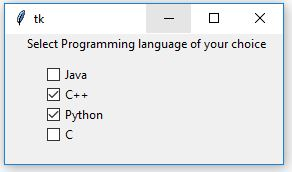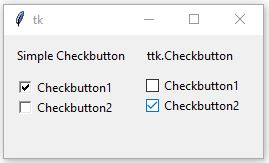# Python | Tkinter ttk.Checkbutton and comparison with simple Checkbutton

Tkinter is a GUI (Graphical User Interface) module which comes along with the Python itself. This module is widely used to create GUI applications. `tkinter.ttk` is used to create the GUI applications with the effects of modern graphics which cannot be achieved using only tkinter. Checkbutton is used to select multiple options.

Checkbuttons can be created using following snippet.

`chkbtn = ttk.Checkbutton(master, option=value, ...)`

Code #1:

 `# importing tkinter.ttk ` `from` `tkinter ``import` `*` `from` `tkinter.ttk ``import` `*`  ` `  `# creating root ` `root ``=` `Tk() ` ` `  `# label text ` `Label(root, text ``=``'Select Programming language of your choice'``).place(x ``=` `20``, y ``=` `0``) ` ` `  `# check buttons ` `java ``=` `Checkbutton(root, text ``=``'Java'``, ` `                   ``takefocus ``=` `0``).place(x ``=` `40``, y ``=` `30``) ` ` `  `cpp ``=` `Checkbutton(root, text ``=``'C++'``, ` `                  ``takefocus ``=` `0``).place(x ``=` `40``, y ``=` `50``) ` ` `  `python ``=` `Checkbutton(root, text ``=``'Python'``, ` `                     ``takefocus ``=` `0``).place(x ``=` `40``, y ``=` `70``) ` ` `  `c ``=` `Checkbutton(root, text ``=``'C'``, ` `                ``takefocus ``=` `0``).place(x ``=` `40``, y ``=` `90``) ` ` `  `root.mainloop() `

Output:Code #2: Difference between simple `Checkbutton` and `ttk.Checkbutton`

 `# importing tkinter and ttk ` `from` `tkinter ``import` `*`  `from` `tkinter ``import` `ttk ` ` `  `# root ` `root ``=` `Tk() ` ` `  `# This will depict the features of Simple Checkbutton ` `Label(root, text ``=``'Simple Checkbutton'``).place(x ``=` `10``, y ``=` `10``) ` `chkbtn1 ``=` `Checkbutton(root, text ``=``'Checkbutton1'``, ` `             ``takefocus ``=` `0``).place(x ``=` `10``, y ``=` `40``) ` `chkbtn2 ``=` `Checkbutton(root, text ``=``'Checkbutton2'``, ` `             ``takefocus ``=` `0``).place(x ``=` `10``, y ``=` `60``) ` ` `  ` `  `# This will depict the features of ttk.Checkbutton ` `Label(root, text ``=``'ttk.Checkbutton'``).place(x ``=` `140``, y ``=` `10``) ` `chkbtn1 ``=` `ttk.Checkbutton(root, text ``=``'Checkbutton1'``, ` `                ``takefocus ``=` `0``).place(x ``=` `140``, y ``=` `40``) ` `chkbtn2 ``=` `ttk.Checkbutton(root, text ``=``'Checkbutton2'``,  ` `                ``takefocus ``=` `0``).place(x ``=` `140``, y ``=` `60``) ` ` `  `root.mainloop() `

Output:Notice the difference in the appearance of both the Checkbuttons which is all due to the modern graphics. In the above Code when mouse is being hovered over the `ttk.Checkbutton` you may see a blue color effect (effect may change from os from os). When we hover mouse over Simple Checkbutton you may not experience any such kind of effects.

My Personal Notes arrow_drop_upCheck out this Author's contributed articles.

If you like GeeksforGeeks and would like to contribute, you can also write an article using contribute.geeksforgeeks.org or mail your article to contribute@geeksforgeeks.org. See your article appearing on the GeeksforGeeks main page and help other Geeks.

Please Improve this article if you find anything incorrect by clicking on the "Improve Article" button below.

Article Tags :

Be the First to upvote.

Please write to us at contribute@geeksforgeeks.org to report any issue with the above content.